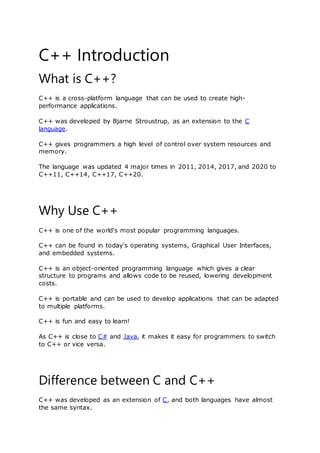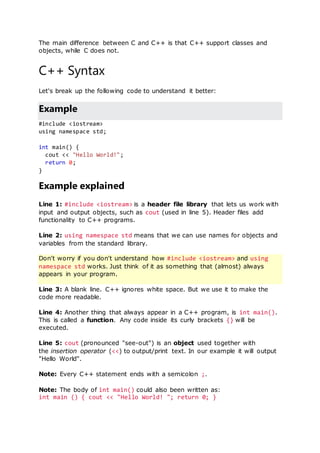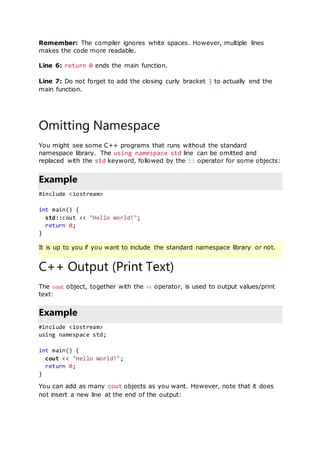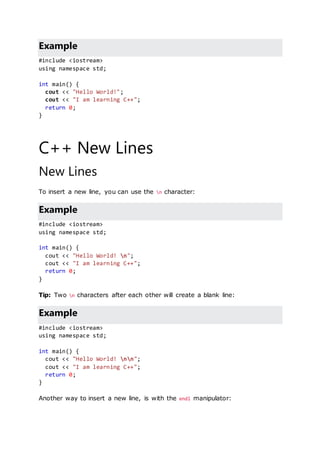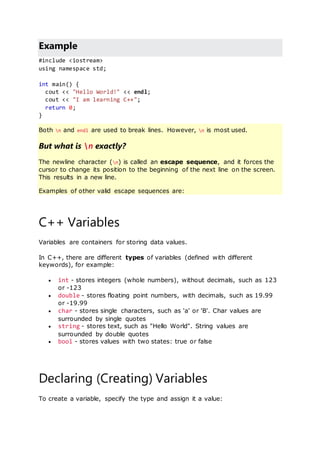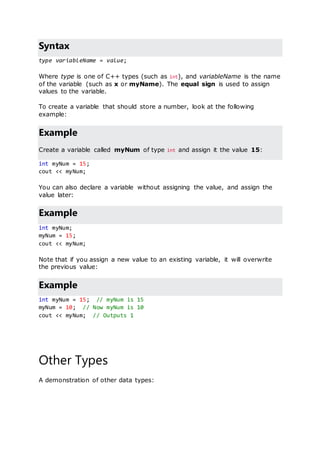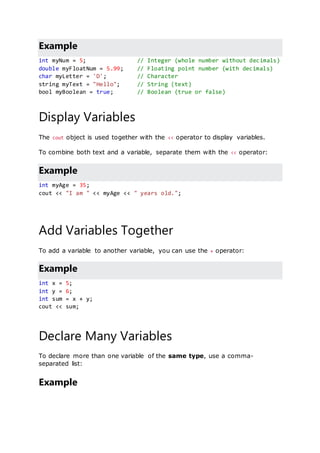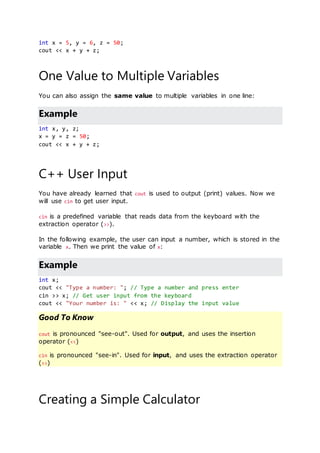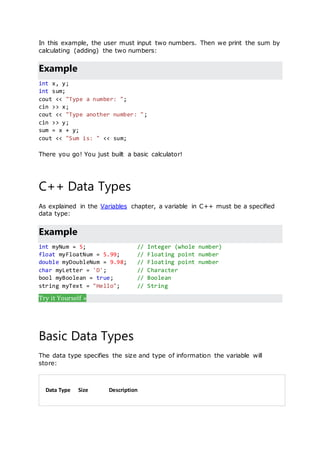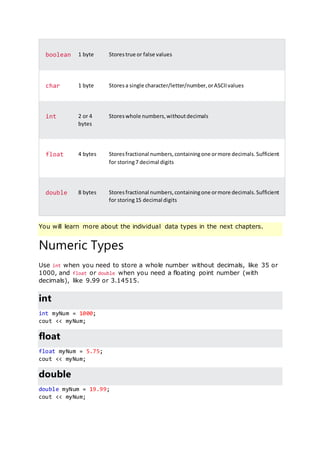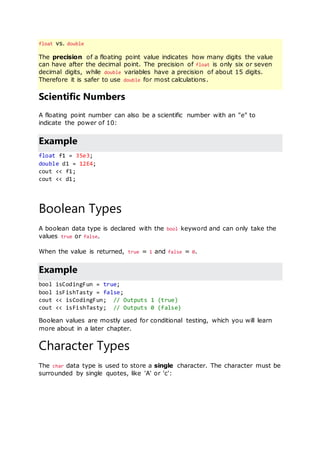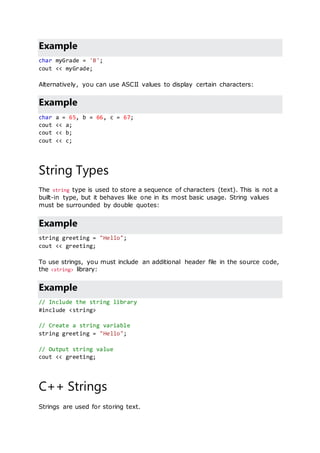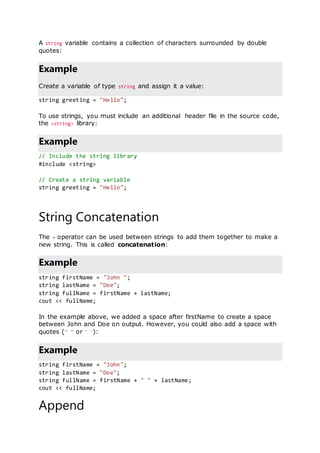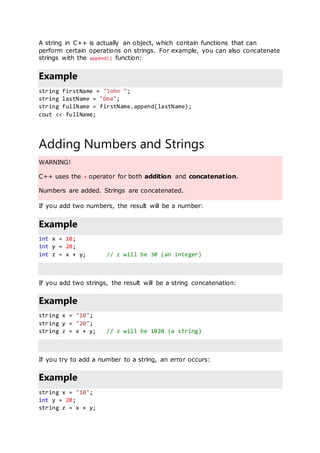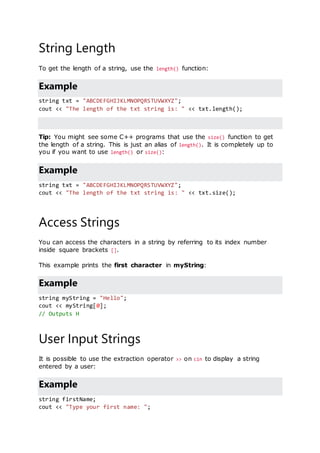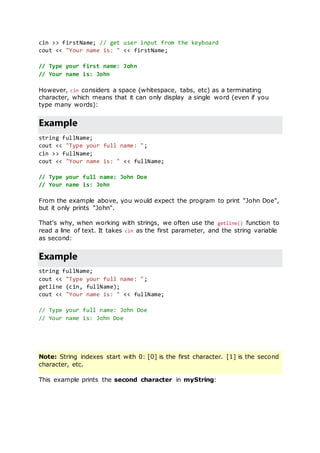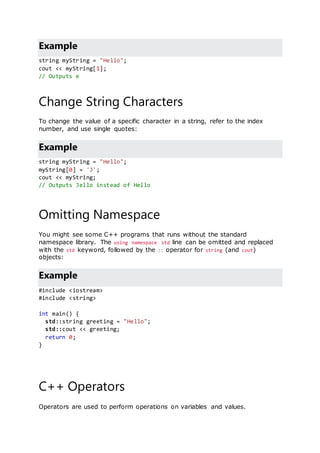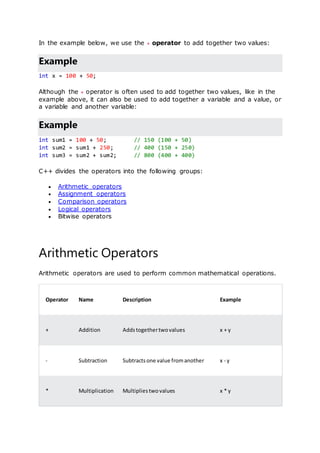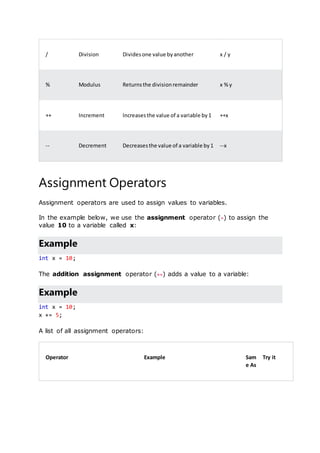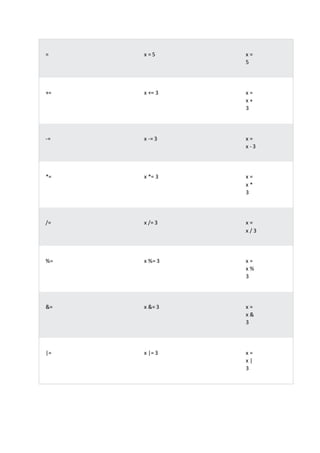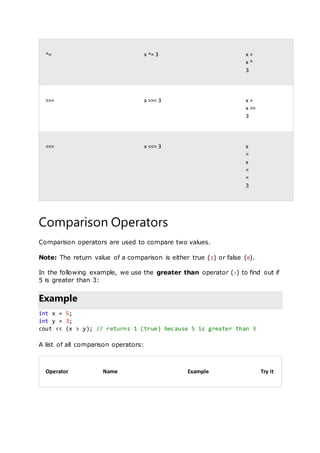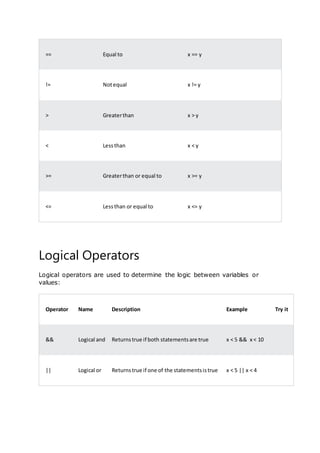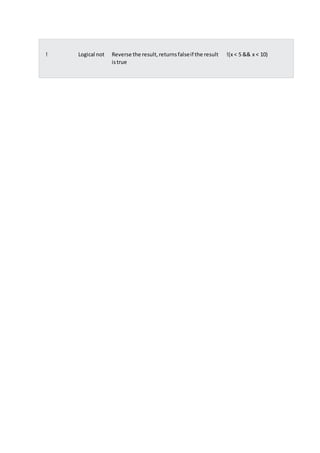Próximo SlideShare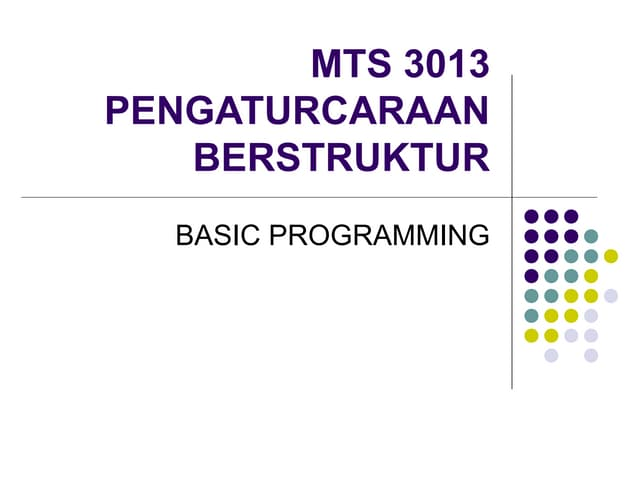Pengaturcaraan asas
Cargando en ... 3
1 de 23

### C++ Tutorial.docx

1. C++ Introduction What is C++? C++ is a cross-platform language that can be used to create high- performance applications. C++ was developed by Bjarne Stroustrup, as an extension to the C language. C++ gives programmers a high level of control over system resources and memory. The language was updated 4 major times in 2011, 2014, 2017, and 2020 to C++11, C++14, C++17, C++20. Why Use C++ C++ is one of the world's most popular programming languages. C++ can be found in today's operating systems, Graphical User Interfaces, and embedded systems. C++ is an object-oriented programming language which gives a clear structure to programs and allows code to be reused, lowering development costs. C++ is portable and can be used to develop applications that can be adapted to multiple platforms. C++ is fun and easy to learn! As C++ is close to C# and Java, it makes it easy for programmers to switch to C++ or vice versa. Difference between C and C++ C++ was developed as an extension of C, and both languages have almost the same syntax.
2. The main difference between C and C++ is that C++ support classes and objects, while C does not. C++ Syntax Let's break up the following code to understand it better: Example #include <iostream> using namespace std; int main() { cout << "Hello World!"; return 0; } Example explained Line 1: #include <iostream> is a header file library that lets us work with input and output objects, such as cout (used in line 5). Header files add functionality to C++ programs. Line 2: using namespace std means that we can use names for objects and variables from the standard library. Don't worry if you don't understand how #include <iostream> and using namespace std works. Just think of it as something that (almost) always appears in your program. Line 3: A blank line. C++ ignores white space. But we use it to make the code more readable. Line 4: Another thing that always appear in a C++ program, is int main(). This is called a function. Any code inside its curly brackets {} will be executed. Line 5: cout (pronounced "see-out") is an object used together with the insertion operator (<<) to output/print text. In our example it will output "Hello World". Note: Every C++ statement ends with a semicolon ;. Note: The body of int main() could also been written as: int main () { cout << "Hello World! "; return 0; }
3. Remember: The compiler ignores white spaces. However, multiple lines makes the code more readable. Line 6: return 0 ends the main function. Line 7: Do not forget to add the closing curly bracket } to actually end the main function. Omitting Namespace You might see some C++ programs that runs without the standard namespace library. The using namespace std line can be omitted and replaced with the std keyword, followed by the :: operator for some objects: Example #include <iostream> int main() { std::cout << "Hello World!"; return 0; } It is up to you if you want to include the standard namespace library or not. C++ Output (Print Text) The cout object, together with the << operator, is used to output values/print text: Example #include <iostream> using namespace std; int main() { cout << "Hello World!"; return 0; } You can add as many cout objects as you want. However, note that it does not insert a new line at the end of the output:
4. Example #include <iostream> using namespace std; int main() { cout << "Hello World!"; cout << "I am learning C++"; return 0; } C++ New Lines New Lines To insert a new line, you can use the n character: Example #include <iostream> using namespace std; int main() { cout << "Hello World! n"; cout << "I am learning C++"; return 0; } Tip: Two n characters after each other will create a blank line: Example #include <iostream> using namespace std; int main() { cout << "Hello World! nn"; cout << "I am learning C++"; return 0; } Another way to insert a new line, is with the endl manipulator:
5. Example #include <iostream> using namespace std; int main() { cout << "Hello World!" << endl; cout << "I am learning C++"; return 0; } Both n and endl are used to break lines. However, n is most used. But what is n exactly? The newline character (n) is called an escape sequence, and it forces the cursor to change its position to the beginning of the next line on the screen. This results in a new line. Examples of other valid escape sequences are: C++ Variables Variables are containers for storing data values. In C++, there are different types of variables (defined with different keywords), for example:  int - stores integers (whole numbers), without decimals, such as 123 or -123  double - stores floating point numbers, with decimals, such as 19.99 or -19.99  char - stores single characters, such as 'a' or 'B'. Char values are surrounded by single quotes  string - stores text, such as "Hello World". String values are surrounded by double quotes  bool - stores values with two states: true or false Declaring (Creating) Variables To create a variable, specify the type and assign it a value:
6. Syntax type variableName = value; Where type is one of C++ types (such as int), and variableName is the name of the variable (such as x or myName). The equal sign is used to assign values to the variable. To create a variable that should store a number, look at the following example: Example Create a variable called myNum of type int and assign it the value 15: int myNum = 15; cout << myNum; You can also declare a variable without assigning the value, and assign the value later: Example int myNum; myNum = 15; cout << myNum; Note that if you assign a new value to an existing variable, it will overwrite the previous value: Example int myNum = 15; // myNum is 15 myNum = 10; // Now myNum is 10 cout << myNum; // Outputs 1 Other Types A demonstration of other data types:
7. Example int myNum = 5; // Integer (whole number without decimals) double myFloatNum = 5.99; // Floating point number (with decimals) char myLetter = 'D'; // Character string myText = "Hello"; // String (text) bool myBoolean = true; // Boolean (true or false) Display Variables The cout object is used together with the << operator to display variables. To combine both text and a variable, separate them with the << operator: Example int myAge = 35; cout << "I am " << myAge << " years old."; Add Variables Together To add a variable to another variable, you can use the + operator: Example int x = 5; int y = 6; int sum = x + y; cout << sum; Declare Many Variables To declare more than one variable of the same type, use a comma- separated list: Example
8. int x = 5, y = 6, z = 50; cout << x + y + z; One Value to Multiple Variables You can also assign the same value to multiple variables in one line: Example int x, y, z; x = y = z = 50; cout << x + y + z; C++ User Input You have already learned that cout is used to output (print) values. Now we will use cin to get user input. cin is a predefined variable that reads data from the keyboard with the extraction operator (>>). In the following example, the user can input a number, which is stored in the variable x. Then we print the value of x: Example int x; cout << "Type a number: "; // Type a number and press enter cin >> x; // Get user input from the keyboard cout << "Your number is: " << x; // Display the input value Good To Know cout is pronounced "see-out". Used for output, and uses the insertion operator (<<) cin is pronounced "see-in". Used for input, and uses the extraction operator (>>) Creating a Simple Calculator
9. In this example, the user must input two numbers. Then we print the sum by calculating (adding) the two numbers: Example int x, y; int sum; cout << "Type a number: "; cin >> x; cout << "Type another number: "; cin >> y; sum = x + y; cout << "Sum is: " << sum; There you go! You just built a basic calculator! C++ Data Types As explained in the Variables chapter, a variable in C++ must be a specified data type: Example int myNum = 5; // Integer (whole number) float myFloatNum = 5.99; // Floating point number double myDoubleNum = 9.98; // Floating point number char myLetter = 'D'; // Character bool myBoolean = true; // Boolean string myText = "Hello"; // String Try it Yourself » Basic Data Types The data type specifies the size and type of information the variable will store: Data Type Size Description
10. boolean 1 byte Storestrue or false values char 1 byte Storesa single character/letter/number,orASCIIvalues int 2 or 4 bytes Storeswhole numbers,withoutdecimals float 4 bytes Storesfractional numbers,containingone ormore decimals.Sufficient for storing7 decimal digits double 8 bytes Storesfractional numbers,containingone ormore decimals.Sufficient for storing15 decimal digits You will learn more about the individual data types in the next chapters. Numeric Types Use int when you need to store a whole number without decimals, like 35 or 1000, and float or double when you need a floating point number (with decimals), like 9.99 or 3.14515. int int myNum = 1000; cout << myNum; float float myNum = 5.75; cout << myNum; double double myNum = 19.99; cout << myNum;
11. float vs. double The precision of a floating point value indicates how many digits the value can have after the decimal point. The precision of float is only six or seven decimal digits, while double variables have a precision of about 15 digits. Therefore it is safer to use double for most calculations. Scientific Numbers A floating point number can also be a scientific number with an "e" to indicate the power of 10: Example float f1 = 35e3; double d1 = 12E4; cout << f1; cout << d1; Boolean Types A boolean data type is declared with the bool keyword and can only take the values true or false. When the value is returned, true = 1 and false = 0. Example bool isCodingFun = true; bool isFishTasty = false; cout << isCodingFun; // Outputs 1 (true) cout << isFishTasty; // Outputs 0 (false) Boolean values are mostly used for conditional testing, which you will learn more about in a later chapter. Character Types The char data type is used to store a single character. The character must be surrounded by single quotes, like 'A' or 'c':
12. Example char myGrade = 'B'; cout << myGrade; Alternatively, you can use ASCII values to display certain characters: Example char a = 65, b = 66, c = 67; cout << a; cout << b; cout << c; String Types The string type is used to store a sequence of characters (text). This is not a built-in type, but it behaves like one in its most basic usage. String values must be surrounded by double quotes: Example string greeting = "Hello"; cout << greeting; To use strings, you must include an additional header file in the source code, the <string> library: Example // Include the string library #include <string> // Create a string variable string greeting = "Hello"; // Output string value cout << greeting; C++ Strings Strings are used for storing text.
13. A string variable contains a collection of characters surrounded by double quotes: Example Create a variable of type string and assign it a value: string greeting = "Hello"; To use strings, you must include an additional header file in the source code, the <string> library: Example // Include the string library #include <string> // Create a string variable string greeting = "Hello"; String Concatenation The + operator can be used between strings to add them together to make a new string. This is called concatenation: Example string firstName = "John "; string lastName = "Doe"; string fullName = firstName + lastName; cout << fullName; In the example above, we added a space after firstName to create a space between John and Doe on output. However, you could also add a space with quotes (" " or ' '): Example string firstName = "John"; string lastName = "Doe"; string fullName = firstName + " " + lastName; cout << fullName; Append
14. A string in C++ is actually an object, which contain functions that can perform certain operations on strings. For example, you can also concatenate strings with the append() function: Example string firstName = "John "; string lastName = "Doe"; string fullName = firstName.append(lastName); cout << fullName; Adding Numbers and Strings WARNING! C++ uses the + operator for both addition and concatenation. Numbers are added. Strings are concatenated. If you add two numbers, the result will be a number: Example int x = 10; int y = 20; int z = x + y; // z will be 30 (an integer) If you add two strings, the result will be a string concatenation: Example string x = "10"; string y = "20"; string z = x + y; // z will be 1020 (a string) If you try to add a number to a string, an error occurs: Example string x = "10"; int y = 20; string z = x + y;
15. String Length To get the length of a string, use the length() function: Example string txt = "ABCDEFGHIJKLMNOPQRSTUVWXYZ"; cout << "The length of the txt string is: " << txt.length(); Tip: You might see some C++ programs that use the size() function to get the length of a string. This is just an alias of length(). It is completely up to you if you want to use length() or size(): Example string txt = "ABCDEFGHIJKLMNOPQRSTUVWXYZ"; cout << "The length of the txt string is: " << txt.size(); Access Strings You can access the characters in a string by referring to its index number inside square brackets []. This example prints the first character in myString: Example string myString = "Hello"; cout << myString; // Outputs H User Input Strings It is possible to use the extraction operator >> on cin to display a string entered by a user: Example string firstName; cout << "Type your first name: ";